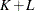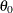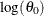# The SEVERITY Procedure

### Scoring Functions

Subsections:

Scoring refers to the act of evaluating a distribution function, such as LOGPDF, SDF, or QUANTILE, on an observation by using the fitted parameter estimates of that distribution. You can do scoring in a DATA step by using the OUTEST= data set that you create with PROC SEVERITY. However, that approach requires some cumbersome programming. In order to simplify the scoring process, you can specify that PROC SEVERITY create scoring functions for each fitted distribution.

As an example, assume that you have fitted the Pareto distribution by using PROC SEVERITY and that it converges. Further assume that you want to use the fitted distribution to evaluate the probability of observing a loss value greater than some set of regulatory limits {L} that are encoded in a data set. You can simplify this scoring process as follows. First, in the PROC SEVERITY step that fits your distributions, you create the scoring functions library by specifying the OUTSCORELIB statement as illustrated in the following steps:

proc severity data=input;
loss lossclaim;
dist pareto;
outscorelib outlib=sasuser.fitdist;
run;


Upon successful completion, if the Pareto distribution model has converged, then the Sasuser.Fitdist library contains the SEV_SDF scoring function in addition to other scoring functions, such as SEV_PDF, SEV_LOGPDF, and so on. Further, PROC SEVERITY also sets the CMPLIB system option to include the Sasuser.Fitdist library. If the set of limits {L} is recorded in the variable Limit in the scoring data set Work.Limits, then you can submit the following DATA step to compute the probability of seeing a loss greater than each limit:

data prob;
set work.limits;
exceedance_probability = sev_sdf(limit);
run;


Without the use of scoring functions, you can still perform this scoring task, but the DATA step that you need to write to accomplish it becomes more complicated and less flexible. For example, you would need to read the parameter estimates from some output created by PROC SEVERITY. To do that, you would need to know the parameter names, which are different for different distributions; this in turn would require you to write a specific DATA step for each distribution or to write a SAS macro. With the use of scoring functions, you can accomplish that task much more easily.

If you fit multiple distributions, then you can specify the COMMONPACKAGE option in the OUTSCORELIB statement as follows:

proc severity data=input;
loss lossclaim;
dist exp pareto weibull;
outscorelib outlib=sasuser.fitdist commonpackage;
run;


The preceding step creates scoring functions such as SEV_SDF_Exp, SEV_SDF_Pareto, and SEV_SDF_Weibull. You can use them to compare the probabilities of exceeding the limit for different distributions by using the following DATA step:

data prob;
set work.limits;
exceedance_exp = sev_sdf_exp(limit);
exceedance_pareto = sev_sdf_pareto(limit);
exceedance_weibull = sev_sdf_weibull(limit);
run;


#### Formal Description

PROC SEVERITY creates a scoring function for each distribution function. A distribution function is defined as any function named dist_suffix, where dist is the name of a distribution that you specify in the DIST statement and the function’s signature is identical to the signature of the required distribution function such as dist_CDF or dist_LOGCDF. For example, for the function 'FOO_BAR' to be a distribution function, you must specify the distribution 'FOO' in the DIST statement and you must define 'FOO_BAR' in the following manner if the distribution 'FOO' has parameters named 'P1' and 'P2':

   function FOO_BAR(y, P1, P2);
/* Code to compute BAR by using y, P1, and P2 */
R = <computed BAR>;
return (R);
endsub;


For more information about the signature that defines a distribution function, see the description of the dist_CDF function in the section Defining a Severity Distribution Model with the FCMP Procedure.

The name and package of the scoring function of a distribution function depend on whether you specify the COMMONPACKAGE option in the OUTSCORELIB statement.

When you do not specify the COMMONPACKAGE option, the scoring function that corresponds to the distribution function dist_suffix is named SEV_suffix, where SEV_ is the standard prefix of all scoring functions. The scoring function is created in a package named dist. Each scoring function accepts only one argument, the value of the loss variable, and returns the same value as the value returned by the corresponding distribution function for the final estimates of the distribution’s parameters. For example, for the preceding 'FOO_BAR' distribution function, the scoring function named 'SEV_BAR' is created in the package named 'FOO' and 'SEV_BAR' has the following signature:

   function SEV_BAR(y);
/* returns value of FOO_BAR for the supplied value
of y and fitted values of P1, P2 */
endsub;


If you specify the COMMONPACKAGE option in the OUTSCORELIB statement, then the scoring function that corresponds to the distribution function dist_suffix is named SEV_suffix_dist, where SEV_ is the standard prefix of all scoring functions. The scoring function is created in a package named sevfit. For example, for the preceding 'FOO_BAR' distribution function, if you specify the COMMONPACKAGE option, the scoring function named 'SEV_BAR_FOO' is created in the sevfit package and 'SEV_BAR_FOO' has the following signature:

   function SEV_BAR_FOO(y);
/* returns value of FOO_BAR for the supplied value
of y and fitted values of P1, P2 */
endsub;


#### Scoring Functions for the Scale Regression Model

If you use the SCALEMODEL statement to specify a scale regression model, then PROC SEVERITY generates the scoring functions when you specify only singleton continuous effects. If you specify interaction or classification effects, then scoring functions are not generated.

For a scale regression model, the estimate of the scale parameter or the log-transformed scale parameter of the distribution depends on the values of the regressors. So PROC SEVERITY creates a scoring function that has the following signature, where x{*} represents the array of regressors:

   function SEV_BAR(y, x{*});
/* returns value of FOO_BAR for the supplied value of x and fitted values of P1, P2 */
endsub;


As an illustration of using this form, assume that you submit the following PROC SEVERITY step to create the scoring library Sasuser.Scalescore:

proc severity data=input;
loss lossclaim;
scalemodel x1-x3;
dist pareto;
outscorelib outlib=sasuser.scalescore;
run;


Your scoring data set must contain all the regressors that you specify in the SCALEMODEL statement. You can submit the following DATA step to score observations by using the scale regression model:

data prob;
array regvals{*} x1-x3;
set work.limits;
exceedance_probability = sev_sdf(limit, regvals);
run;


PROC SEVERITY creates two utility functions, SEV_NUMREG and SEV_REGNAME, in the OUTLIB= library that return the number of regressors and name of a given regressor, respectively. They are described in detail in the next section. These utility functions are useful when you do not have easy access to the regressor names in the SCALEMODEL statement. You can use the utility functions as follows:

data prob;
array regvals{10} _temporary_;
set work.limits;
do i = 1 to sev_numreg();
regvals(i) = input(vvaluex(sev_regname(i)), best12.);
end;
exceedance_probability = sev_sdf(limit, regvals);
run;


The dimension of the regressor values array that you supply to the scoring function must be equal to, where K is the number of regressors that you specify in the SCALEMODEL statement irrespective of whether PROC SEVERITY deems any of those regressors to be redundant. L is 1 if you specify an OFFSET= variable in the SCALEMODEL statement, and 0 otherwise.

#### Utility Functions and Subroutines in the OUTLIB= Library

In addition to creating the scoring functions for all distribution functions, PROC SEVERITY creates the following utility functions and subroutines in the OUTLIB= library.

SEV_NUMPARM | SEV_NUMPARM_dist

is a function that returns the number of distribution parameters and has the following signature:

• Type: Function

• Number of arguments: 0

• Sequence and type of arguments: Not applicable

• Return value: Numeric value that contains the number of distribution parameters

If you do not specify the COMMONPACKAGE option in the OUTSCORELIB statement, then a function named SEV_NUMPARM is created in the package of each distribution. Here is a sample structure of the code that PROC SEVERITY uses to define the function:

    function SEV_NUMPARM();
n = <number of distribution parameters>;
return (n);
endsub;


If you specify the COMMONPACKAGE option in the OUTSCORELIB statement, then for each distribution dist, the function named SEV_NUMPARM_dist is created in the sevfit package. SEV_NUMPARM_dist has the same structure as the SEV_NUMPARM function that is described previously.

SEV_PARMEST | SEV_PARMEST_dist

is a subroutine that returns the estimate and standard error of a specified distribution parameter and has the following signature:

• Type: Subroutine

• Number of arguments: 3

• Sequence and type of arguments:

index

specifies the numeric value of the index of the distribution parameter for which you want the information. The value of index must be in the interval [1,m], where m is the number of parameters in the distribution to which this subroutine belongs.

est

specifies the output argument that returns the estimate of the requested parameter.

stderr

specifies the output argument that returns the standard error of the requested parameter.

• Return value: Estimate and standard error of the requested distribution parameter that are returned in the output arguments est and stderr, respectively

If you do not specify the COMMONPACKAGE option in the OUTSCORELIB statement, then a subroutine named SEV_PARMEST is created in the package of each distribution. Here is a sample structure of the code that PROC SEVERITY uses to define the subroutine:

    subroutine SEV_PARMEST(index, est, stderr);
outargs est, stderr;
est = <value of the estimate for the distribution parameter
at position 'index'>;
stderr =  <value of the standard error for distribution parameter
at position 'index'>;
endsub;


If you specify the COMMONPACKAGE option in the OUTSCORELIB statement, then for each distribution dist, the subroutine named SEV_PARMEST_dist is created in the sevfit package. SEV_PARMEST_dist has the same structure as the SEV_PARMEST subroutine that is described previously.

If you use the SCALEMODEL statement to specify a scale regression model, and if you specify only singleton continuous effects, then for index=1, the returned estimates are of, the base value of the scale parameter, orif the distribution has a log-scale parameter. For more information about, see the section Estimating Regression Effects.

SEV_PARMNAME | SEV_PARMNAME_dist

is a function that returns the name of a specified distribution parameter and has the following signature:

• Type: Function

• Number of arguments: 1

• Sequence and type of arguments:

index

specifies the numeric value of the index of the distribution parameter for which you want the information. The value of index must be in the interval [1,m], where m is the number of parameters in the distribution to which this function belongs.

• Return value: Character value that contains the name of the distribution parameter that appears at the position index in the distribution’s definition

If you do not specify the COMMONPACKAGE option in the OUTSCORELIB statement, then a function named SEV_PARMNAME is created in the package of each distribution. Here is a sample structure of the code that PROC SEVERITY uses to define the function:

    function SEV_PARMNAME(index) $32; name = <name of the distribution parameter at position 'index'>; return (name); endsub;  If you specify the COMMONPACKAGE option in the OUTSCORELIB statement, then for each distribution dist, a function named SEV_PARMNAME_dist is created in the sevfit package. SEV_PARMNAME_dist has the same structure as the SEV_PARMNAME function that is described previously. If you use the SCALEMODEL statement to specify a scale regression model, and if you specify only singleton continuous effects, then the following helper functions and subroutines are also created in the OUTLIB= library. SEV_NUMREG is a function that returns the number of regressors and has the following signature: • Type: Function • Number of arguments: 0 • Sequence and type of arguments: Not applicable • Return value: Numeric value that contains the number of regressors that you specify in the SCALEMODEL statement. If you specify an OFFSET= variable in the SCALEMODEL statement, then the returned value is equal to 1 plus the number of regressors that you specify in the SCALEMODEL statement. Here is a sample structure of the code that PROC SEVERITY uses to define the function:  function SEV_NUMREG(); m = <number of regressors>; if (<offset variable is specified>) then m = m + 1; return (m); endsub;  This function does not depend on any distribution, so it is always created in the sevfit package. SEV_REGEST | SEV_REGEST_dist is a subroutine that returns the estimate and standard error of a specified regression parameter and has the following signature: • Type: Subroutine • Number of arguments: 3 • Sequence and type of arguments: index specifies the numeric value of the index of the regression parameter for which you want the information. The value of index must be in the interval [1,K], where K is the number of regressors as returned by the SEV_NUMREG function. If you specify an OFFSET= variable in the SCALEMODEL statement, then an index value of K corresponds to the offset variable. est specifies the output argument that returns the estimate of the requested regression parameter. stderr specifies the output argument that returns the standard error of the requested regression parameter. • Return value: Estimate and standard error of the requested regression parameter that are returned in the output arguments est and stderr, respectively If you do not specify the COMMONPACKAGE option in the OUTSCORELIB statement, then a subroutine named SEV_REGEST is created in the package of each distribution. Here is a sample structure of the code that PROC SEVERITY uses to define the subroutine:  subroutine SEV_REGEST(index, est, stderr); outargs est, stderr; est = <value of the estimate for the regression parameter at position 'index'>; stderr = <value of the standard error for regression parameter at position 'index'>; endsub;  If you specify the COMMONPACKAGE option in the OUTSCORELIB statement, then for each distribution dist, the subroutine named SEV_REGEST_dist is created in the sevfit package. SEV_REGEST_dist has the same structure as the SEV_REGEST subroutine that is described previously. If the regressor that corresponds to the specified index value is a redundant regressor, the returned values of both est and stderr are equal to the special missing value of .R. If you specify an OFFSET= variable in the SCALEMODEL statement and if the index value corresponds to the offset variable—that is, it is equal to the value that the SEV_NUMREG function returns — then the returned value of est is equal to 1 and the returned value of stderr is equal to the special missing value of .F. SEV_REGNAME is a function that returns the name of a specified regressor and has the following signature: • Type: Function • Number of arguments: 1 • Sequence and type of arguments: index specifies the numeric value of the index of the regressor for which you want the name. The value of index must be in the interval [1,K], where K is the number of regressors as returned by the SEV_NUMREG function. If you specify an OFFSET= variable in the SCALEMODEL statement, then an index value of K corresponds to the offset variable. • Return value: Character value that contains the name of the regressor that appears at the position index in the SCALEMODEL statement. If you specify an OFFSET= variable in the SCALEMODEL statement, then for an index value of K, the returned value contains the name of the offset variable. Here is a sample structure of the code that PROC SEVERITY uses to define the function:  function SEV_REGNAME(index)$32;
name = <name of regressor at position 'index'>;
return (name);
endsub;


This function does not depend on any distribution, so it is always created in the sevfit package.